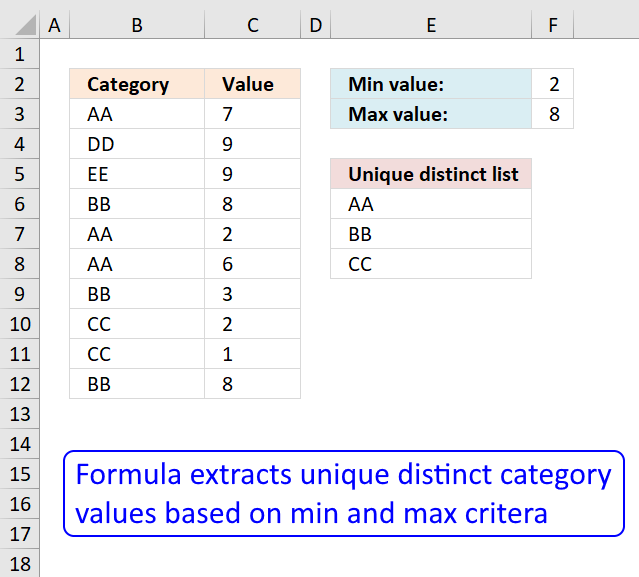Author: Oscar Cronquist Article last updated on November 20, 2018The regular formula in cell E6 extracts unique distinct values from column B based on the corresponding number in column C, the min value is in cell F2 and the Max value is in cell F3.

Formula in cell E6:

=LOOKUP(2, 1/((COUNTIF(\$E\$5:E5, \$B\$3:\$B\$12)=0)*(\$C\$3:\$C\$12<=\$F\$3)*(\$C\$3:\$C\$12>=\$F\$2)), \$B\$3:\$B\$12)

### Explaining formula in cell E6

#### Step 1 - Prevent duplicate values

The COUNTIF function counts values based on a condition, in this case, multiple conditions. The first argument has an expanding cell range that grows when the cell is copied to cells below. This allows the formula to count for previously shown values.

COUNTIF(\$E\$5:E5, \$B\$3:\$B\$12)=0

becomes

COUNTIF("Unique distinct list",{"AA";"DD";"EE";"BB";"AA";"AA";"BB";"CC";"CC";"BB"})=0

becomes

{0;0;0;0;0;0;0;0;0;0}=0

and returns

{TRUE;TRUE;TRUE;TRUE;TRUE;TRUE;TRUE;TRUE;TRUE;TRUE}.

#### Step 2 - Check which records meet criteria

The less than and greater than signs lets you create logical expressions. Each condition is checked against the values in column C.

(\$C\$3:\$C\$12<=\$F\$3)*(\$C\$3:\$C\$12>=\$F\$2)

becomes

{7;9;9;8;2;6;3;2;1;8}<=8)*({7;9;9;8;2;6;3;2;1;8}>=2)

becomes

({TRUE;FALSE;FALSE;TRUE;TRUE;TRUE;TRUE;TRUE;TRUE;TRUE})*({TRUE;TRUE;TRUE;TRUE;TRUE;TRUE;TRUE;TRUE;FALSE;TRUE})

Both arguments must be TRUE, we must then multiply the arrays.

{1;0;0;1;1;1;1;1;0;1}

#### Step 3 - Multiply arrays

All criteria must be met.

(COUNTIF(\$E\$5:E5,\$B\$3:\$B\$12)=0)*(\$C\$3:\$C\$12<=\$F\$3)*(\$C\$3:\$C\$12>=\$F\$2)

becomes

{TRUE;TRUE;TRUE;TRUE;TRUE;TRUE;TRUE;TRUE;TRUE;TRUE}*{1;0;0;1;1;1;1;1;0;1}

and returns

{1;0;0;1;1;1;1;1;0;1}

#### Step 4 - Divide 1 with array

The LOOKUP function ignores errors, if we divide something with 0 (zero) we get #DIV/0! error.

1/((COUNTIF(\$E\$5:E5,\$B\$3:\$B\$12)=0)*(\$C\$3:\$C\$12<=\$F\$3)*(\$C\$3:\$C\$12>=\$F\$2))

becomes

1/{1;0;0;1;1;1;1;1;0;1}

and returns

{1;#DIV/0!;#DIV/0!;1;1;1;1;1;#DIV/0!;1}.

#### Step 5 - Return value

LOOKUP(2, 1/((COUNTIF(\$E\$5:E5, \$B\$3:\$B\$12)=0)*(\$C\$3:\$C\$12<=\$F\$3)*(\$C\$3:\$C\$12>=\$F\$2)), \$B\$3:\$B\$12)

becomes

LOOKUP(2, {1;#DIV/0!;#DIV/0!;1;1;1;1;1;#DIV/0!;1}, \$B\$3:\$B\$12)

becomes

LOOKUP(2, {1;#DIV/0!;#DIV/0!;1;1;1;1;1;#DIV/0!;1}, {"AA";"DD";"EE";"BB";"AA";"AA";"BB";"CC";"CC";"BB"})